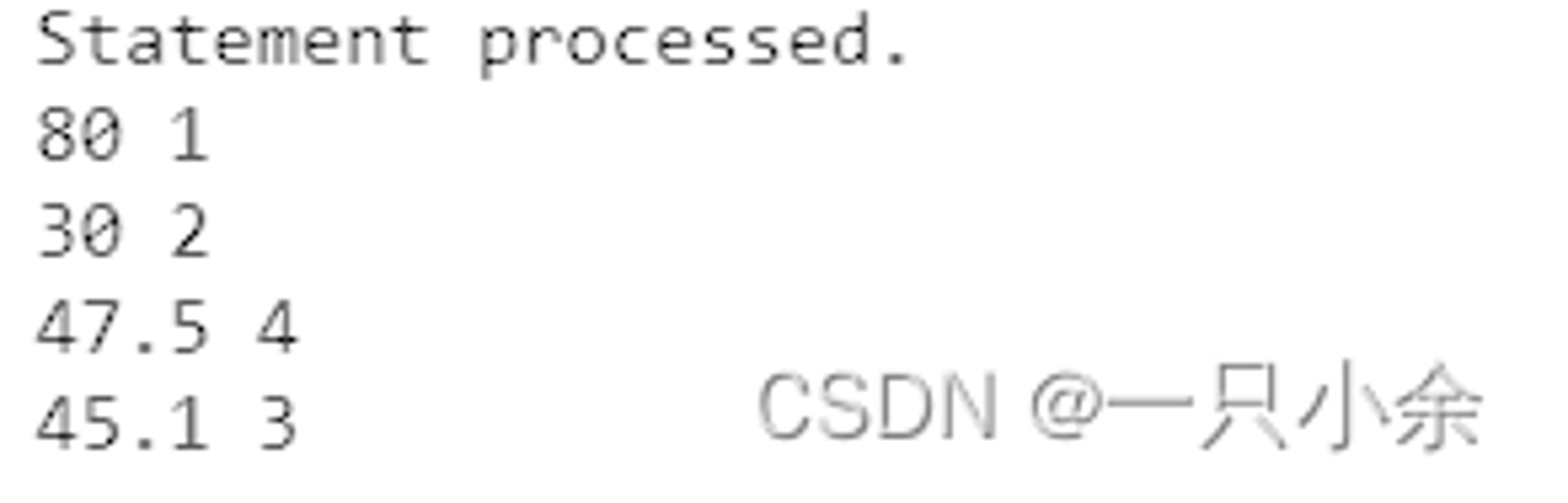V1

2023/02/13阅读：19主题：科技蓝

# 基础

## PL/SQL结构

1.声明 2.执行 3.异常

``declare 变量名字 类型;begin 程序运行exception when 异常 then  处理end;``

## 语法

``````不区别大小写

所有大小写字母
数字
空白符
符号：+-*等
``````

+,-, *, / 加法，减法/负，乘法，除法
% 游标属性绑定或代表任意字符的通配符
' 字符串分隔符
. 从属关系
, 表达式或列表分隔符
: 主机变量指示符
" 引用标识符分隔符
= 关系等
@ 数据库链接符
; 声明或语句终止符
:= 赋值运算符
=> 位置定位符
ΙΙ 字符串连接运算符
** 指数运算符
<<, >> 标签分隔符(开始和结束)
/*, */ 多行注释分隔符(开始和结束)
-- 单行注释指示符
.. 范围运算符
<, >, <=, >= 关系运算符
<>, '=, ~=, ^= 不同版本的”不等于”运算符

``````1.字符型
2.数字
3.布尔
4.日期
``````

``````单行 --

``````

``````每一行只能定义一个变量
constant代表常量必须赋初值
not null 关键字不行赋初值
:= / default 赋初值
``````

# sql语句

## SELECT语句

select into语句 注意这个语句一次只能记录一个信息 如果没有查到则会发生NO_DATA_FOUND异常 如果查到多个则会产生TOO_MANY_ROWS异常 查询b员工的信息和id为109员工的名字

``declare v_emp emp%rowtype; v_name emp.name%type;begin select * into v_emp from emp where name='b'; dbms_output.put_line(v_emp.id||' '||v_emp.salary); select name into v_name from emp where id = 109; dbms_output.put_line(v_name);end;``

## DML语句

pl/sql中对dml语句进行了扩展，允许使用变量

# 控制结构

## 选择判断

### if

``if 条件 then 语句;elsif 条件2 then 语句2;else 语句3;end if;``

### case 语句

``case when 条件 then ..; when ... then ...;end case;``

## 循环

### 简单循环

``loop .....; exit [when 退出条件];end loop;``

### while

``while 进入条件 loop .....;end loop;``

### for

``for 循环变量 in {reverse} 下界..上界 .....;end loop;``

1.循环变量不需要显示的定义 2.系统默认变量从下界到上界，如果使用reverse 则从上界到下界递减 3.循环变量只能在循环用

``//普通语句declare v_count binary_integer :=1; v_sum number :=0;begin loop  if mod(v_count,2)=0 then   v_sum:=v_sum+v_count;  end if;  v_count := v_count + 1;  exit when v_count > 100; end loop; dbms_output.put_line(v_sum);end;``
``declare v_count binary_integer :=1; v_sum number :=0;begin while v_count <=100 loop  if mod(v_count,2)=0 then   v_sum:=v_sum+v_count;  end if;  v_count := v_count + 1; end loop; dbms_output.put_line(v_sum);end;``
`` declare v_sum number :=0;begin for v_count in 1..100 loop  if mod(v_count,2)=0 then   v_sum:=v_sum+v_count;  end if; end loop;    dbms_output.put_line(v_sum);end;``

## 跳转结构

``````goto实现
<<>>标记
goto 标记;

pl/sql内部可以跳转，内可以跳到外，外不能跳到内
``````

## 输入

``````输入可以在定义阶段和代码阶段来进行,通过&符号来表示

``````

## 输出

`` dbms_output.put_line(输出的内容)；``

# 游标

``````前面我们知道了select into一次只能查询一条语句。

``````

``````游标分为：
显示游标：用户定义，多行select查询
隐式游标：系统自动，DML和单行select查询
``````

## 显示游标

### 定义游标

`` cursor 名字 is select查询``

1.必须在声明部分定义 2.定义时可以引用PL/SQL变量，但必须在游标定义前 3.定义时没有生成数据，只是保存在数据字典中。 4.可以使用名字%rowtype 定义记录类型的变量。

### 打开游标

`` open 变量名``

1.只有打开游标才会，查询 2.一旦打开就无法再次打开，除非关闭 3.如果游标定义中变量值发生改变，则只能在下次打开才能其作用

### 检索游标/遍历

`` fetch 游标名 into 行名``

### 关闭游标

`` close 名``

### 游标属性

%ISOPEN 布尔 游标是否打开
%FOUND 布尔 判断最近一次使用fetch语句是否有数据
%NOTFOUND 布尔 判断最近一次是否没有数据，没有返回true
%ROWCOUNT 数值 检索个数
%BULK_ROWCOUNT 数值 第i给元素所影响的行数

### 循环检索实例

``declare cursor c_dep IS select d_id,avg(salary) avgsfrom emps group by d_id; v_dep c_dep%rowtype;begin open c_dep; loop  fetch c_dep into v_dep;  exit when c_dep%notfound;  dbms_output.put_line(v_dep.avgs||' '||v_dep.d_id); end loop; closse c_dep;end; ``while循环

``declare cursor c_dep IS select d_id,avg(salary) avgsfrom emps group by d_id; v_dep c_dep%rowtype;begin open c_dep; while c_dep%found loop  fetch c_dep into v_dep;  dbms_output.put_line(v_dep.avgs||' '||v_dep.d_id); end loop; closse c_dep;end; ``

for 循环 for循环会自动打开检索和关闭游标

``declare cursor c_dep IS select d_id,avg(salary) avgsfrom emps group by d_id;begin for v_dep in c_dep loop  dbms_output.put_line(v_dep.avgs||' '||v_dep.d_id); end loop;end; ``

``begin for v_dep in (select d_id,avg(salary) avgsfrom emps group by d_id) loop  dbms_output.put_line(v_dep.avgs||' '||v_dep.d_id); end loop;end; ``

## 隐式游标

### 属性

SQL%isopen 布尔 是否打开
SQL%found 布尔 判断是否对数据库产生影响，如果插入、删除、修改或者查到数据则true
SQL%notfound 布尔 判断是否没有产生影响
SQL%rowcount 布尔 影响行数

## 游标变量

### 定义

``type 名字 is ref cursor [return 类型]``

``名字 引用类型名字；``

### 打开游标变量

``open 游标 for select的sql；``

### 检索游标

`` loop  fetch 名 into ...;  exit when 名%notfound； end loop；``

### 关闭游标

``close 名；``

# 异常

## 异常概述

too_mangy_rows ora-01422 返回多行数据
no_data_found ora-01403 没有发现数据
zero_divide ora-01476 除数为0

## 异常处理

##### 异常定义
``异常变量 exception;``

##### 异常抛出
``raise 异常;``
##### 异常捕获
``exception when 异常 then 处理; ...;end;``

sqlcode 当前错误码
sqlerrm 错误文本

# 例题

1.要求根据输入的不同表名进行不同处理，若表名为employees，则显示高于10号部门平均工资的员工信息。若表名为departments，则显示各个部门的人数。

``declare v_table char(20); type t_cursor is ref cursor; v_cursor t_cursor; v_emp employees%rowtype; v_deptno employees.department_id%type; v_num number;begin v_table:='\$table_name'; if v_table = 'employees' then  open v_cursor for select * from employees where salary > (   select avg(salary) from employees where department_id =10);  while v_cursor%notfound loop   fetch v_cursor into v_emp;   dbms_output.put_line(v_emp....);  end loop elseif v_table ='departments' then  open v_emp for select count(*) num ,department_id from employees group by department_id;  while v_cursor%notfound loop   fetch v_cursor into v_num,v_deptno;   dbms_output.put_line(v_deptno||' '||v_num);  end loop else  raise_application_error(-20000,'Input must be ''emp'' or ''dept'''); end if close v_cursorend;``V1